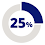# Finding the area of a triangle

The goal of this post is to give you several methods for finding the area of a triangle and a video that goes with each method. The method used to find the area is based on the information you are given.

If you know the Base and Altitude use      half base times height

If you don’t know the altitude    use  Heron’s formula

If you know Two sides and included angle     use Side Angle Side ( SAS)

If you know X and Y vertices of the corners  use Box Method

If you know Triangle is equilateral   use    Equilateral Triangle Area

If you know Triangle is isosceles     use    ½ base times height

Video Half base * height

For even more information on finding the area of a triangle please see.Finding the area of a triangle

#### 1 comment:

1.With all of those methods, there should be no problem finding the area of this triangle:

http://fivetriangles.blogspot.com/2012/04/ratio-problem.html